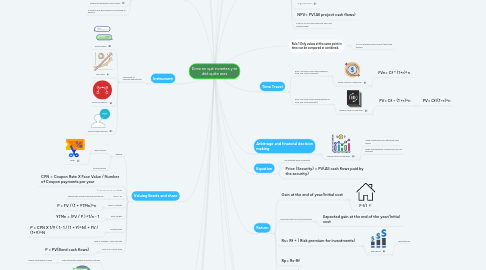# Dime en qué inviertes y te diré quién eresLuis Alfredo Rangel Lappe
Get Started. It's FreeDime en qué inviertes y te diré quién eres## 3. Time Travel

### 3.1. Rule 2 To move a cash flow forward in time, you must compund it.

3.1.1. Future Value of a cash flow.

3.1.1.1. FVn= Cf * (1+r)^n

### 3.2. Rule 3 To move a cash flow backward in time, you must discount it.

3.2.1. Present Value of cash flow.

3.2.1.1. PV= Cf ÷ (1 +r)^n

3.2.1.1.1. PV= Cf/(1÷r)^n

## 5. Compounding

### 5.1. number of time periods increases the future value increases.

5.1.1. Co + Cf1 * (1+r)^1 + Cf2 * (1+r)^2 + --- + Cfn + (1+r)^n

### 5.3. Increasing rate since there is more interest on interest

5.3.1. Co + Cf1/(1+r)^1 + Cf2/(1+r)^n --- + Cfn/(1+r)^n

## 6. Net Present Value NPV

6.3.1. Cf/r

### 6.4. + = GOOD

6.4.1. Accepting or rejecting a project

6.5.1. Choosing among project

## 7. Arbitrage and financial decision making

### 7.1. Valuing costs and benefits

7.1.1. Using market prices to determine cash values

7.1.2. When competetitive market prices are not available

## 10. Instrument

### 10.1. Instrument vs Potential theoretician

10.1.1. Profit bubble

10.1.3. POSITIVE Rumors

## 11. Return

11.1.1. F-I/I

### 11.2. Expected return of a risk investment

11.2.1. Expected gain at the end of the year/Initial cost

### 11.3. Rs= Rf + ( Risk premium for investments)

11.3.1. Extra profit

## 13. Using the payback rule

### 13.1. Payback period the lengt of time required to recover the cost of an investment.

13.1.1. Payback Period = Cost of project / Annual Cash flows

### 13.2. IRR

13.2.1. Internal rate of return

13.2.1.1. try and failure

13.2.1.2. Evalute and compare projects

13.2.1.3. Iterations

## 14. Valuing Bonds and share

### 14.1. Bounds

14.1.1. Zero coupon

14.1.1.1. Cetes

14.1.2. Coupon Bonds

### 14.4. Price = PV

14.4.1. Interest rate at which we discount bonds

### 14.5. Yield to Maturity

14.5.1. P = FV / (1 + YTMn)^n

### 14.6. Zero coupon

14.6.1. YTMn = (FV / P ) ^1/n - 1

### 14.7. Coupon Bond

14.7.1. P = CPN X 1/Y ( 1- 1 / (1 + Y)^N) + FV / (1+Y)^N

### 14.9. Price of a coupon Bond

14.9.1. P = PV(Bond cash flows)

### 15.1. AAA & AA

15.1.1. High credit quality

### 15.2. A & BBB

15.2.1. Medium credit quaility

### 15.3. BB, B & CCC

15.3.1. Low credit quality (junk bonds)

15.4.1. Div / Po

### 15.7. With different dividends

15.7.1. Po = Div1 / 1 + re + Div2 + p2 / (1 + Re)^2 + Divn / (1 + Re)^n

### 15.8. Dividend - Discount Model

15.8.1. Po = Div1 / Re - g

15.8.1.1. G

15.8.1.1.1. Growth rate

## 16. Capital Markets and CAPM (capital asset pricing model)

### 16.1. Small stocks the highest long-terms returns

16.1.1. largest fluctuations in price

### 16.3. T-Bills the lowest long-terms returns

16.3.1. lowest fluctuations in price

### 16.4. Expected return

16.4.1. E (R) = {r Pr X R

### 16.5. Varance

16.5.1. Var (R) = E [(R-E[R])^2]

### 16.6. Volality

16.6.1. Measure the total risk

16.6.1.1. SD ( R) = √ VAR(R)

### 16.8. Beta

16.8.1. measure the systematic risk

16.8.1.1. For every 1% change in the market, how much your security moves.

16.8.1.2. If the B is negative, you move to the inverse of the market

### 16.9. CAPM

16.9.1. Calculate the expected return for a risky security

16.9.2. Calculate the cost of particular project

## 20. Market portofolio

### 20.1. An efficent consists of a weighted sum of each asset in the market

20.2.1. E [Rmkt] - Rf

### 20.3. CAPM

20.3.1. E(R)= rf + B x (E[Rmkt] - rf

## 21. EVA

### 21.1. Measure the value company generates from funds invested

21.1.1. Evan= Cn - r1n-1 - (Depreciation in Period n)

21.1.2. VA = Actual Value

21.1.3. Profitabily Index

21.1.3.1. Value created / Resource Consumed

21.1.3.1.1. NPV / Resource consumed

21.1.4. EVA for a year